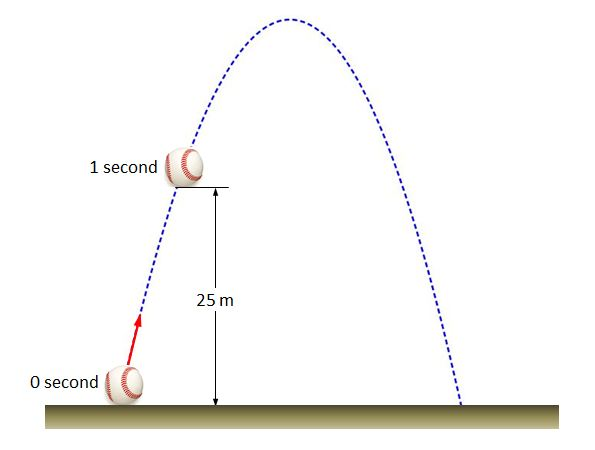# Projectile motion of baseballWhen you throw a baseball obliquely in the air, it shows a projectile motion as in the picture. Suppose that the height of the ball $1$ second after you throw it is $25$ m. Then how many seconds will it take for the ball to reach the peak, from the moment you throw the ball?

Gravitational acceleration is $10 \text{m/s}^2$, and air resistance is negligible.

×